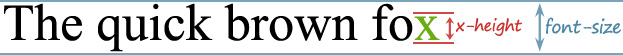CSS BASIC
CSS BOX MODEL
CSS3 FEATURES
CSS3 EXAMPLES
CSS3 REFERENCE

# CSS Units

Units are used to specify non-zero length value in CSS properties.

## Understanding CSS Units

The units in which length is measured can be either absolute such as pixels, points and so on, or relative such as percentages (`%`) and `em` units.

Specifying CSS units is obligatory for non-zero values, because there is no default unit. Missing or ignoring a unit would be treated as an error. However, if the value is 0, the unit can be omitted (after all, zero pixels is the same measurement as zero inches).

Note: Lengths refer to distance measurements. A length is a measurement comprising a numeric value and a unit only such as `10px`, `2em`, `50%` etc. The whitespace can't appear between the number and the unit.

## Relative Length Units

Relative length units specify a length relative to another length property. Relative units are:

Unit Description
em the current font-size
ex the x-height of the current font

The `em` and `ex` units depend on the font size that's applied to the element.

## Using the Em Unit

A measurement of `1em` is equal to the computed value of the `font-size` property of the element on which it is used. It may be used for vertical or horizontal measurement.

For example, if `font-size` of the element set to 16px and `line-height` set to 2.5em then the calculated value of `line-height` in pixel is `2.5 x 16px = 40px`.

#### Example

Try this code »
``````p {
font-size: 16px;
line-height: 2.5em;
}``````

The exception occurs when em is specified in the value of `font-size` property itself, in that case it refers to the font size of the parent element.

So, when we specify a font size in `em`, 1em is equal to the inherited `font-size`. As such, `font-size: 1.2em;` makes the text 1.2 times larger than the parent element's text.

#### Example

Try this code »
``````body {
font-size: 62.5%;
font-family: Arial, Helvetica, sans-serif;
}
p {
font-size: 1.6em;
}
p:firt-letter {
font-size: 3em;
font-weight: bold;
}``````

Let's understand what this code was all about. The default size for the fonts in all modern browsers is 16px. Our first step is to reduce this size for the entire document by setting the body `font-size` to 62.5%, which resets the font-size to 10px (62.5% of 16px).

This is to round off the default `font-size` for easy `px` to `em` conversion.

## Using the Ex Unit

The `ex` unit is equal to the x-height of the current font.

The x-height is so called because it is often equal to the height of the lowercase 'x', as illustrated below. However, an `ex` is defined even for fonts that do not contain an 'x'.## Absolute Length Units

Absolute length units are fixed in relation to each other. They are highly dependent on the output medium, so are mainly useful when the output environment is known. The absolute units consist of the physical units (`in`, `cm`, `mm`, `pt`, `pc`) and the `px` unit.

Unit Description
`in` inches – 1in is equal to 2.54cm.
`cm` centimeters.
`mm` millimeters.
`pt` points – In CSS, one point is defined as 1/72 inch (0.353mm).
`pc` picas – 1pc is equal to 12pt.
`px` pixel units – 1px is equal to 0.75pt.

Absolute physical units such as `in`, `cm`, `mm`, etc. should be used for print media and similar high-resolution devices. Whereas, for on-screen display such as desktop and lower-resolution devices, it is recommended to use the pixel or em units.

#### Example

Try this code »
``````h1 { margin: 0.5in; }       /* inches  */
h2 { line-height: 3cm; }    /* centimeters */
h3 { word-spacing: 4mm; }   /* millimeters */
h4 { font-size: 12pt; }     /* points */
h5 { font-size: 1pc; }      /* picas */
h6 { font-size: 12px; }     /* picas */``````

Tip: Style sheets that use the relative units such as em or percentage (%) can more easily scale from one output environment to another.